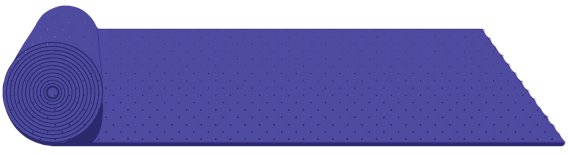Carpet Rolling 101A carpet is rolled into the form of a cylinder (by rolling it along the length) of radius $\frac{27}{140}\text{ m}$ and it is kept on a rough horizontal floor. The carpet is given a negligible (gentle) push and it starts to unroll without slipping. Calculate the horizontal velocity in $\text{m}/\text{s}$ of the axis of the cylindrical part of the carpet when its radius becomes $\frac{27}{280}$.

Note: Approximate $g$ to be $10 \text{ m}/\text{s}^2.$

×